# Branching point (of a minimal surface)

(diff) ← Older revision | Latest revision (diff) | Newer revision → (diff)

A singular point of a minimal surface in which the first fundamental form of the surface vanishes; this means, in fact, that such a branching point can exist on a generalized minimal surface only. This singular point owes its name to the fact that in a neighbourhood of it the structure of the generalized minimal surface resembles that of the Riemann surface of the function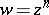,, over the point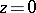, i.e. there the generalized minimal surface has a many-sheeted orthogonal projection onto some plane domain, in which the projection of the branching point itself is an interior point with a unique pre-image. In a neighbourhood of a branching pointthe coordinates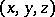of the minimal surface can be represented in the form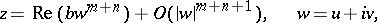whereand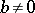are two complex constants,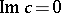;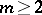, and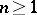are integers named, respectively, the order and the index of the singular point and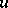and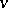are intrinsic isothermal coordinates.

The following theorem was deduced on the basis of this representation: If the numbers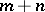andare coprime, then the minimal surface has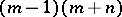different lines of self-intersection issuing from the singular point in different directions. There is a relation between the genus of a complete minimal surface, the number of its branching points, and the index of its Gaussian mapping .

One distinguishes between two kinds of branching points: false branching points and true (non-false) branching points. False branching points are singularities of the mapping which defines the surface that can be got rid of by re-parametrization (e.g. if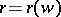is a regular minimal surface, then the generalized minimal surfacehas a false branching point at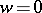). A true branching point represents a real singularity of the surface itself, and has the following important property: In a neighbourhood of a true branching point the surface can be altered so that the new surface which coincides with the original one outside the deformed neighbourhood will have a smaller area than the original surface (this holds for surfaces in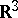but is not true in more-general settings: e.g. for the area-minimizing surfaces in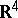). The theory of generalized minimal surfaces with a branching point served as a base of the general theory of branched immersions, developed for a broad class of two-dimensional surfaces in,, .

How to Cite This Entry:
Branching point (of a minimal surface). Encyclopedia of Mathematics. URL: http://encyclopediaofmath.org/index.php?title=Branching_point_(of_a_minimal_surface)&oldid=17524
This article was adapted from an original article by I.Kh. Sabitov (originator), which appeared in Encyclopedia of Mathematics - ISBN 1402006098. See original article